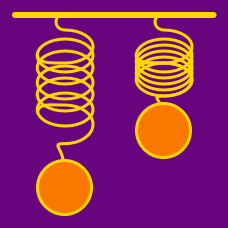Classical Mechanics

# Linear restoring force - perturbation analysis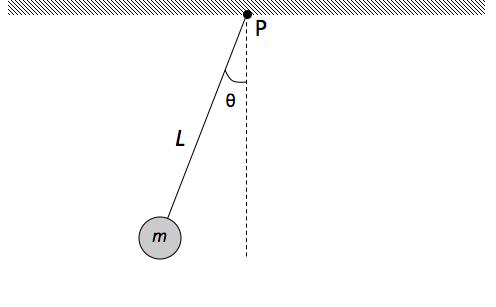As shown in the figure above, a physical pendulum consists of a disc of radius $R = 5.0 \text{ cm}$ and mass $m = 1.0 \text{ kg}$ fixed at the end of a massless rod. The other end of the rod is pivoted about point $P$ on the ceiling. The distance from the pivot point to the center of mass of the bob is $L =4 \text{ m}.$ Initially the bob is released from rest from a small angle $\theta_0$ with respect to the vertical. Find the period of the bob.

Assumptions and Details

• The gravitational acceleration is $g = 9.8 \text{ m/s}^2.$

What is the frequency of a simple pendulum with arm length $l = 2.00 \text{ m}$ that is in an elevator accelerating upward at a rate of $a = 1.00 \text{ m/s}^2$?

Assumptions and Details

• Assume that the amplitude of the simple pendulum is very small.
• The gravitational acceleration is $g = 9.80 \text{ m/s}^2.$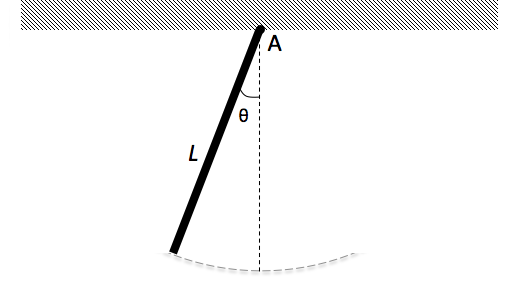A physical pendulum consists of a $6.0 \text{ m}$ long stick with mass $m = 100 \text{ g}$ joined to the ceiling, as shown in the above figure. What is the pendulum’s period of oscillation about point $A$ at the tip of the stick?

Assumptions and Details

• The gravitational acceleration is $g= 9.8 \text{ m/s}^2.$
• Assume that the amplitude of the pendulum is very small.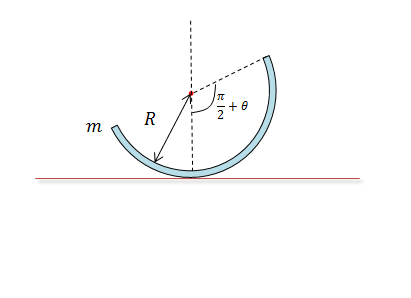There is a half-ring of density $\rho = 4 \text{ kg/m}$ and radius $R = 2 \text{ m}$. When it is perturbed by small angle $\theta$, it oscillates. If the ring's period can be expressed as $T = 2\pi\sqrt{\frac{a}{b}} \text{ s},$ where $a$ and $b$ are coprime positive integers, what is the value of $a+b?$

The gravitational acceleration is $g=10\text{ m/s}^2.$
Assume that $\sqrt{2} = 1.5$ and $\pi = 3 .$ (This is a model of a roly-poly.)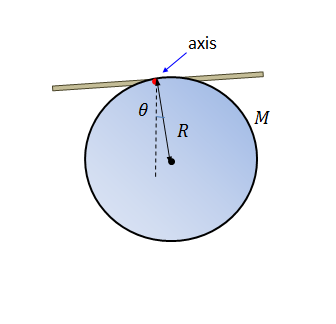A disk of mass $M = 1 \text{ kg}$ and radius $R = 4 \text{ m}$ oscillates on a rod. If the disk's period can be expressed as $T = 2\pi\sqrt{\frac{a}{b}} \text{ s},$ where $a$ and $b$ are coprime positive integers, what is the value of $a+b?$

Assume that $\theta$ is very small.
The moment of inertia on the axis is given by $I = \frac{3}{2}MR^2.$
The gravitational acceleration is $g=10\text{ m/s}^2.$

×